## RS Aggarwal Class 7 Solutions Chapter 6 Algebraic Expressions Ex 6A

These Solutions are part of RS Aggarwal Solutions Class 7. Here we have given RS Aggarwal Solutions Class 7 Chapter 6 Algebraic Expressions Ex 6A.

Other Exercises

Question 1.
Solution: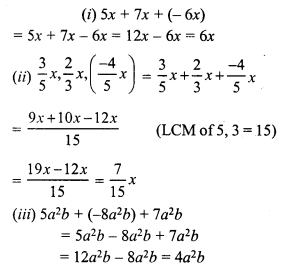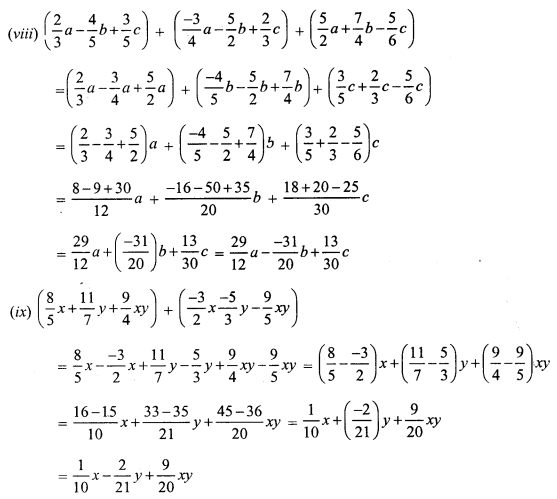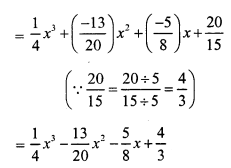Question 2.
Solution:Question 3.
Solution:
Sum of (a + 3b – 4c), (4a – b + 9c) and (-2b + 3c – a)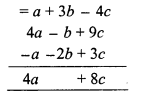Now subtract (2a – 3b + 4c) from 4a + 8c
= 4a + 8c – (2a – 3b + 4c)
= 4a + 8c – 2a + 3b – 4c
= 4a – 2a + 3b + 8c – 4c
= 2a + 3b + 4c

Question 4.
Solution: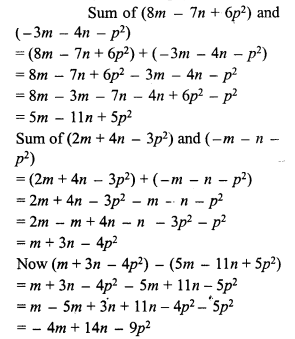Question 5.
Solution:
Sum of (8a – 6a² + 9) and (-10a – 8 + 8a²)
= 8a – 6a² + 9 + (-10a) – 8 + 8a²
= 8a – 10a – 6a² + 8a² + 9 – 8
= -2a + 2a² + 1
Now -3 – (-2a + 2a² + 1)
= (-3 + 2a – 2a² – 1)
= -4 + 2a – 2a²

Question 6.
Solution: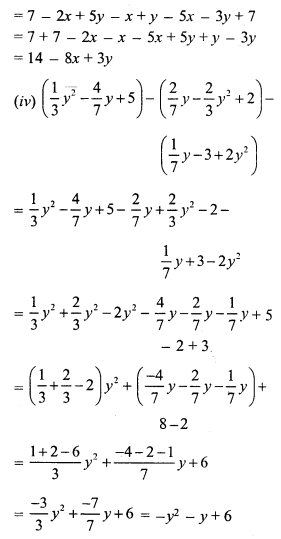Hope given RS Aggarwal Solutions Class 7 Chapter 6 Algebraic Expressions Ex 6A are helpful to complete your math homework.

If you have any doubts, please comment below. Learn Insta try to provide online math tutoring for you.